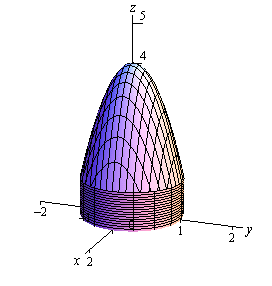Paul's Online Notes
Home / Calculus III / Surface Integrals / Divergence Theorem
Show Mobile Notice Show All Notes Hide All Notes
Mobile Notice
You appear to be on a device with a "narrow" screen width (i.e. you are probably on a mobile phone). Due to the nature of the mathematics on this site it is best views in landscape mode. If your device is not in landscape mode many of the equations will run off the side of your device (should be able to scroll to see them) and some of the menu items will be cut off due to the narrow screen width.

### Section 17.6 : Divergence Theorem

In this section we are going to relate surface integrals to triple integrals. We will do this with the Divergence Theorem.

#### Divergence Theorem

Let $$E$$ be a simple solid region and $$S$$ is the boundary surface of $$E$$ with positive orientation. Let $$\vec F$$ be a vector field whose components have continuous first order partial derivatives. Then,

$\iint\limits_{S}{{\vec F\centerdot d\vec S}} = \iiint\limits_{E}{{{\mathop{\rm div}\nolimits} \vec F\,dV}}$

Let’s see an example of how to use this theorem.

Example 1 Use the divergence theorem to evaluate $$\displaystyle \iint\limits_{S}{{\vec F\centerdot d\vec S}}$$ where $$\vec F = xy\,\vec i - \frac{1}{2}{y^2}\,\vec j + z\,\vec k$$ and the surface consists of the three surfaces, $$z = 4 - 3{x^2} - 3{y^2}$$, $$1 \le z \le 4$$ on the top, $${x^2} + {y^2} = 1$$, $$0 \le z \le 1$$ on the sides and $$z = 0$$ on the bottom.
Show Solution

Let’s start this off with a sketch of the surface.The region $$E$$ for the triple integral is then the region enclosed by these surfaces. Note that cylindrical coordinates would be a perfect coordinate system for this region. If we do that here are the limits for the ranges.

$\begin{array}{c}0 \le z \le 4 - 3{r^2}\\ 0 \le r \le 1\\ 0 \le \theta \le 2\pi \end{array}$

We’ll also need the divergence of the vector field so let’s get that.

${\mathop{\rm div}\nolimits} \vec F = y - y + 1 = 1$

The integral is then,

\begin{align*}\iint\limits_{S}{{\vec F\centerdot d\vec S}} & = \iiint\limits_{E}{{{\mathop{\rm div}\nolimits} \vec F\,dV}}\\ & = \int_{{\,0}}^{{\,2\pi }}{{\int_{{\,0}}^{{\,1}}{{\int_{{\,0}}^{{4 - 3{r^2}}}{{r\,dz}}\,dr}}\,d\theta }}\\ & = \int_{{\,0}}^{{\,2\pi }}{{\int_{{\,0}}^{{\,1}}{{4r - 3{r^3}\,dr}}\,d\theta }}\\ & = \int_{{\,0}}^{{\,2\pi }}{{\left. {\left( {2{r^2} - \frac{3}{4}{r^4}} \right)} \right|_0^1\,d\theta }}\\ & = \int_{{\,0}}^{{\,2\pi }}{{\frac{5}{4}\,d\theta }}\\ & = \frac{5}{2}\pi \end{align*}